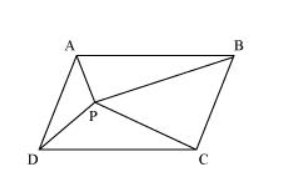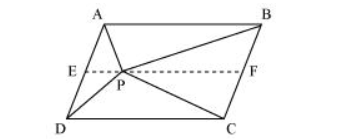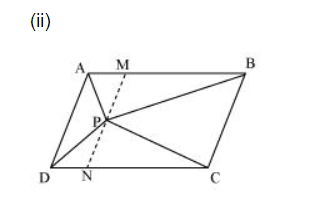# In the given figure, P is a point in the interior of a parallelogram ABCD.

Question. In the given figure, P is a point in the interior of a parallelogram ABCD. Show that

(i) $\operatorname{ar}(A P B)+\operatorname{ar}(P C D)=\frac{1}{2} \operatorname{ar}(A B C D)$

(ii) $\operatorname{ar}(A P D)+\operatorname{ar}(P B C)=\operatorname{ar}(A P B)+\operatorname{ar}(P C D)$Solution:(i) Let us draw a line segment EF, passing through point $P$ and parallel to line segment $A B$.

In parallelogram $A B C D$,

$A B \| E F$ (By construction) ... (1)

ABCD is a parallelogram.

$\therefore A D \| B C$ (Opposite sides of a parallelogram)

$\Rightarrow \mathrm{AE} \| \mathrm{BF} \ldots(2)$

From equations (1) and (2), we obtain

$A B \| E F$ and $A E \| B F$

Therefore, quadrilateral ABFE is a parallelogram.

It can be observed that $\triangle A P B$ and parallelogram $A B F E$ are lying on the same base $A B$ and between the same parallel lines $A B$ and $E F$.

$\therefore$ Area $(\triangle \mathrm{APB})=\frac{1}{2}$ Area $(\mathrm{ABFE})$..(3)

Similarly, for $\triangle P C D$ and parallelogram EFCD,

Area $(\triangle \mathrm{PCD})=\frac{1}{2}$ Area $(\mathrm{EFCD})$..(4)

Adding equations $(3)$ and $(4)$, we obtain

Area $(\Delta \mathrm{APB})+$ Area $(\Delta \mathrm{PCD})=\frac{1}{2}[$ Area $(\mathrm{ABFE})+$ Area $(\mathrm{EFCD})]$

Area $(\Delta \mathrm{APB})+$ Area $(\Delta \mathrm{PCD})=\frac{1}{2}$ Area $(\mathrm{ABCD})$..(5)Let us draw a line segment MN, passing through point $P$ and parallel to line segment $A D$.

In parallelogram $A B C D$,

MN $\| A D$ (By construction) $\ldots$ (6)

ABCD is a parallelogram.

$\therefore A B \| D C$ (Opposite sides of a parallelogram)

$\Rightarrow \mathrm{AM} \| \mathrm{DN} \ldots(7)$

From equations $(6)$ and $(7)$, we obtain

MN $\| A D$ and $A M \| D N$

Therefore, quadrilateral AMND is a parallelogram.

It can be observed that $\triangle A P D$ and parallelogram AMND are lying on the same base AD and between the same parallel lines AD and MN.

$\therefore$ Area $(\triangle \mathrm{APD})=\frac{1}{2}$ Area (AMND) ...(8)

Similarly, for $\triangle \mathrm{PCB}$ and parallelogram MNCB,

Area $(\triangle \mathrm{PCB})=\frac{1}{2}$ Area $(\mathrm{MNCB})$..(9)

Adding equations (8) and (9), we obtain

Area $(\Delta \mathrm{APD})+$ Area $(\Delta \mathrm{PCB})=\frac{1}{2}[$ Area $(\mathrm{AMND})+$ Area $(\mathrm{MNCB})]$

Area $(\Delta \mathrm{APD})+$ Area $(\Delta \mathrm{PCB})=\frac{1}{2}$ Area $(\mathrm{ABCD})$..(10)

On comparing equations $(5)$ and $(10)$, we obtain

Area $(\Delta \mathrm{APD})+$ Area $(\Delta \mathrm{PBC})=$ Area $(\Delta \mathrm{APB})+$ Area $(\Delta \mathrm{PCD})$